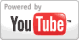Multiplying Decimals - Pre Algebra Lesson
Grade: 4 - 8 | In this math lesson, learn to multiply decimals in the same way that one would multiply whole numbers. At the end, determine where the decimal goes in the answer.Add to My DashboardMultiplication
Math Games & Activities :
GradesMath Games      (with special printable formats for classroom use)
1st - 5th Multiply by Grouping, #1Multiply by Grouping, #2Multiply by Adding
Times Tables :     of 2    of 3    of 4    of 5    of 6    of 7    of 8    of 9    of 10    of 11    of 12
Missing Factors : of 2    of 3    of 4    of 5    of 6    of 7    of 8    of 9    of 10    of 11    of 12
Times Tables, Quiz #1Times Tables, Quiz #2Times Tables, Quiz #3
Multiply Doubles       Multiply Tens       Multiply HundredsMultiply 2-digit x 1-digit
Multiplication : Word Problems, #1    Word Problems, #2Multiply 3-digit x 1-digit### Search Videos & Games